# DAV Class 5 Maths Chapter 3 Worksheet 5 Solutions

The DAV Class 5 Maths Book Solutions Pdf and DAV Class 5 Maths Chapter 3 Worksheet 5 Solutions of Multiples and Factors offer comprehensive answers to textbook questions.

## DAV Class 5 Maths Ch 3 Worksheet 5 Solutions

Question 1.
Answer the following questions. The first one is done for you.
(a) Is 5 a factor of 36?
Solution:
No, 36 ÷ 5 = 7 and remainder = 1

(b) Is 7 a factor of 77?
Solution:
Yes; 77 ÷ 7 = 11 and Remainder = 0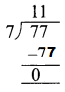(c) Is 8 a factor of 62?
Solution:
No; 62 ÷ 8 = 7 and remainder = 6(d) Is 9 a factor of 70?
Solution:
No; 70 ÷ 9 = 7 and remainder = 7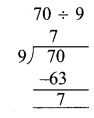(e) Is 12 a factor of 120?
Solution:
Yes; 120 ÷ 12 = 10 remainder = 0(f) Is 15 a factor of 100?
Solution:
No; 100 ÷ 15 = 6 and remainder = 10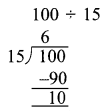(g) Is 20 a factor of 140?
Solution:
Yes; 140 ÷ 20 = 7 and remainder = 0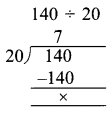(h) Is 6 a factor of 284?
Solution:
No; 284 ÷ 6 = 47 and remainder = 2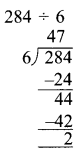Question 2.
Fill in the blanks.
(a) 2 × 7 = 14 : 2 and ____ are the factors of 14.
Solution:
1

(b) 3 × 8 = 24 : ____ and 8 are factors of 24.
Solution:
3

(c) 5 × 7 = 35 : 5 and 7 are the factors of _____
Solution:
35

(d) 4 × 5 = 20 : 4 and 5 are the _____ of 20.
Solution:
factors

(e) 7 × 8 = 56 : 7 and ____ are the _____ of 56.
Solution:
8; factorsQuestion 3.
Write all the factors of the following numbers.
(a) 12
Solution:
Factors of 12 = 1, 2, 3, 4, 6, 12(b) 32
Solution:
Factors of 32 = 1, 2, 4, 8, 16, 32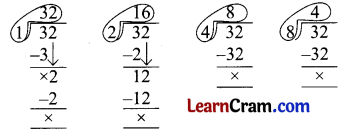(c) 28
Solution:
Factors of 28 = 1, 2, 4, 7, 14, 28(d) 35
Solution:
Factors of 35 = 1, 5, 7, 35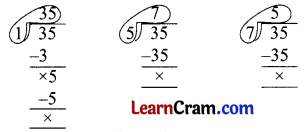(e) 45
Solution:
Factors of 45 = 1, 3, 5, 9, 15, 45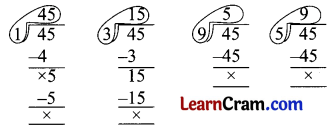(f) 60
Solution:
Factors of 60 = 1, 2, 3, 4, 5, 6, 10, 12, 15, 20, 30, 60.(g) 50
Solution:
Factors of 50 = 1, 2, 5, 10, 25, 50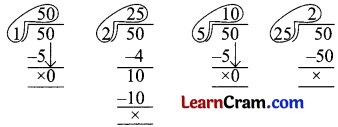(h) 72
Solution:
Factors of 72 = 1, 2, 3, 4, 6, 8, 9, 12, 18, 24, 36, 72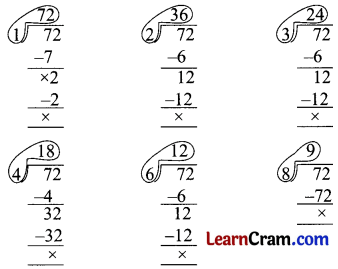(i) 96
Solution:
Factors of 96 = 1, 2, 3, 4, 6, 8, 12, 16, 24, 32, 48, 96(j) 84
Solution:
Factors of 84 = 1, 2, 3, 4, 6, 7, 12, 14, 21, 28, 42, 84(k) 88
Solution:
Factors of 88 = 1, 2, 4, 8, 11, 22, 44, 88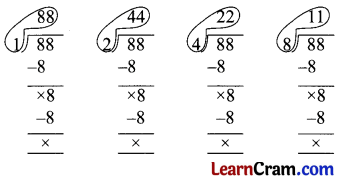(l) 71
Solution:
Factors of 71 = 1, 71DAV Class 5 Maths Chapter 3 Worksheet 5 Notes

If we divide 12 apples into different groups.
Each group should have an equal number of apples.
No apples should be left out.
Each grouping should be different.
Multiplication ⇒ 1 × 12 = 12
2 × 6 = 12
3 × 4 = 12
4 × 3 = 12
6 × 2 = 12
12 × 1 = 12
12 apples can be arranged in different groups having 12, 6, 4, 3, 2, and 1 apples.
1, 2, 3, 4, 6, and 12 are called the factors of 12.
If we divide 12 by each of its factors, the remainder will be zero.To find the factors of a number, we divide the number by 1, 2, 3, 4, 5, 6, 7,……..Example 1.
Find all the factors of 18
Solution: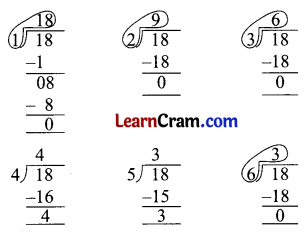Stop here as the same multiplication fact
3 × 6 = 18
6 × 3 = 18
So factors of 18 are 1 and 18; 2 and 9; 3 and 6. Thus 1, 2, 3, 6, 9, and 18 are factors of 18.
Another method: Multiplication facts of 18So the factors of 18 are 1 and 18; 2 and 9; 3 and 6.
Or
1, 2, 3, 6, and 18 are the factors of 18.
1 is a factor of every number.
Every number is a factor of itself.